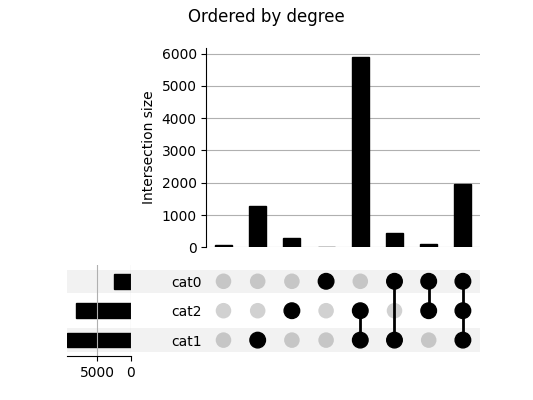# UpSetPlot documentation¶This is another Python implementation of UpSet plots by Lex et al. [Lex2014]. UpSet plots are used to visualise set overlaps; like Venn diagrams but more readable. Documentation is at https://upsetplot.readthedocs.io.

This upsetplot library tries to provide a simple interface backed by an extensible, object-oriented design.

There are many ways to represent the categorisation of data, as covered in our Data Format Guide.

Our internal input format uses a pandas.Series containing counts corresponding to subset sizes, where each subset is an intersection of named categories. The index of the Series indicates which rows pertain to which categories, by having multiple boolean indices, like example in the following:

>>> from upsetplot import generate_counts
>>> example = generate_counts()
>>> example
cat0   cat1   cat2
False  False  False      56
True      283
True   False    1279
True     5882
True   False  False      24
True       90
True   False     429
True     1957
Name: value, dtype: int64


Then:

>>> from upsetplot import plot
>>> plot(example)
>>> from matplotlib import pyplot
>>> pyplot.show()


makes:And you can save the image in various formats:

>>> pyplot.savefig("/path/to/myplot.pdf")
>>> pyplot.savefig("/path/to/myplot.png")


This plot shows the cardinality of every category combination seen in our data. The leftmost column counts items absent from any category. The next three columns count items only in cat1, cat2 and cat3 respectively, with following columns showing cardinalities for items in each combination of exactly two named sets. The rightmost column counts items in all three sets.

## Rotation¶

We call the above plot style “horizontal” because the category intersections are presented from left to right. Vertical plots are also supported!## Distributions¶

Providing a DataFrame rather than a Series as input allows us to expressively plot the distribution of variables in each subset.While the dataset above is randomly generated, you can prepare your own dataset for input to upsetplot. A helpful tool is from_memberships, which allows us to reconstruct the example above by indicating each data point’s category membership:

>>> from upsetplot import from_memberships
>>> example = from_memberships(
...     [[],
...      ['cat2'],
...      ['cat1'],
...      ['cat1', 'cat2'],
...      ['cat0'],
...      ['cat0', 'cat2'],
...      ['cat0', 'cat1'],
...      ['cat0', 'cat1', 'cat2'],
...      ],
...      data=[56, 283, 1279, 5882, 24, 90, 429, 1957]
... )
>>> example
cat0   cat1   cat2
False  False  False      56
True      283
True   False    1279
True     5882
True   False  False      24
True       90
True   False     429
True     1957
dtype: int64


See also from_contents, another way to describe categorised data, and from_indicators which allows each category to be indicated by a column in the data frame (or a function of the column’s data such as whether it is a missing value).

### Installation¶

To install the library, you can use pip:

\$ pip install upsetplot


Installation requires:

It should then be possible to:

>>> import upsetplot


in Python.

### Why an alternative to py-upset?¶

Probably for petty reasons. It appeared py-upset was not being maintained. Its input format was undocumented, inefficient and, IMO, inappropriate. It did not facilitate showing plots of each subset’s distribution as in Lex et al’s work introducing UpSet plots. Nor did it include the horizontal bar plots illustrated there. It did not support Python 2. I decided it would be easier to construct a cleaner version than to fix it.